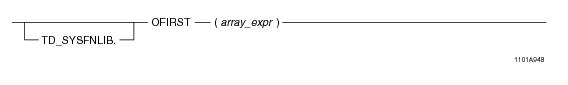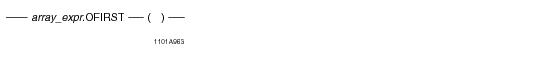# 15.00 - OFIRST - Teradata Database

## Teradata Database SQL Functions, Operators, Expressions, and Predicates

Product
Release Number
15.00
Content Type
Programming Reference
Publication ID
B035-1145-015K
Language
English (United States)

## OFIRST

### Purpose

Returns the lowest subscript value in array_expr as either an unsigned INTEGER value or a new instance of the predefined ARRAY type ArrayVec.

### Syntax

System function syntax:Method-style syntax:where:

 Syntax element… Specifies… TD_SYSFNLIB the name of the database where the method is located. array_expr an ARRAY expression, which is one of the following: A reference to an ARRAY column An ARRAY constructor expression. See “ARRAY Constructor Expression” on page 195. A UDF expression A UDM expression

### ANSI Compliance

This is a Teradata extension to the ANSI SQL:2011 standard.

### Usage Notes

OFIRST takes an array expression as an argument and returns the lowest subscript value in the ARRAY type. If array_expr is a one-dimensional ARRAY type, OFIRST returns an unsigned INTEGER value. If array_expr is a multidimensional ARRAY type, OFIRST returns a new instance of the predefined ARRAY type ArrayVec, containing the subscript information for the first element in the array. If the array is empty (all elements of the array are in an uninitialized state), then OFIRST returns NULL.

If array_expr is NULL, an error is returned.

The OFIRST method is compatible with the Oracle FIRST method for one-dimensional ARRAY types. However, the empty set of parentheses required by Teradata syntax is a deviation from Oracle syntax.

### Result Type

OFIRST returns an unsigned INTEGER value or a new instance of the predefined ARRAY type ArrayVec.

### Example

Consider the following one-dimensional ARRAY data type and table.

`CREATE TYPE phonenumbers AS VARRAY(20) OF CHAR(10);`
` `
`CREATE TABLE employee_info (eno INTEGER, phonelist phonenumbers);`

The table is populated with the following values:

`/* The first 2 elements are populated; the rest are uninitialized. */`
`INSERT INTO employee_info VALUES (1, `
`   phonenumbers('1112223333', '6195551234'));`
` `
`/* Empty ARRAY instance */`
`INSERT INTO employee_info VALUES (2, `
`   phonenumbers());`

The following query returns the lowest subscript value in the phonelist array.

`SELECT eno, phonelist.OFIRST()`
`FROM employee_info;`

The following is the result of the query.

`ENO         phonelist.OFIRST()`
`---         ------------------`
` 1           1`
` 2           1`

### Example

Consider the following 2-D ARRAY data type and table.

`CREATE TYPE shot_ary AS VARRAY(1:50)(1:50) OF INTEGER;`
` `
`CREATE TABLE seismic_table (`
`   id INTEGER,`
`   shots shot_ary);`

The table is populated with the following values:

`/* The first 2 elements are populated; the rest are uninitialized. */`
`INSERT INTO seismic_table VALUES (1, shot_ary(11, 12));`
` `
`/* Empty ARRAY instance */`
`INSERT INTO seismic_table VALUES (2, shot_ary());`

The following query returns the lowest subscript value in the shots array.

`SELECT id, shots.OFIRST()`
`FROM seismic_table;`

The following is the result of the query.

`ID          shots.OFIRST()`
`--          --------------`
` 1           NEW arrayVec(1,1)`
` 2           NEW arrayVec(1,1)`

The following is the same query using function-style syntax.

`SELECT id, OFIRST(shots) `
`FROM seismic_table;`